American Journal of Modern Energy
Volume 2, Issue 6, December 2016, Pages: 43-47

Critical Point Symmetry, X (5), in 154Gd

Salah A. Eid1, Sohair M. Diab2

1Physics Department, Faculty of Engineering, Ain Shams University, Cairo, Egypt

2Physics Department, Faculty of Education, Ain Shams University, Cairo, Egypt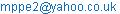(S. A. Eid)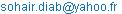(S. M. Diab)

Salah A. Eid, Sohair M. Diab. Critical Point Symmetry, X (5), in 154Gd. American Journal of Modern Energy. Vol. 2, No. 6, 2016, pp. 43-47. doi: 10.11648/j.ajme.20160206.12

Received: September 29, 2016; Accepted: October 28, 2016; Published: November 21, 2016

Abstract: The positive-negative parity states, potential energy surfaces, V(β, γ), transition probabilities, B(E1), B(E2), staggering effect and electric monopole strength, X (E0/E2), values of 154Gd have been calculated within the frame work of the interacting boson approximation model (I BA 1). The results obtained are compared to the available experimental, theoretical data and reasonable agreement has achieved. The potential energy surfaces, levels energy and transition probability ratios show that 154Gd is an X (5) candidate.

Keywords: Levels Energy, Transition Probability, B(E1), B(E2), Electric Monopole Strength, X (E0/E2)

Contents

1. Introduction

The even-even 154Gd isotope has subjected to an extensive study in the past few years where the region of N= 90 shows rapid changes of nuclear deformation parameters as a function of the particle number. Many authors have studied this isotope experimentally and theoretically.

Experimentally [1-10] different techniques were applied. Silicon and germanium detector arrays, (α, 2n), (α, 4n) reactions, AFRODITE γ-ray spectrometer, combination of γ -ray scattering experiments and γ - γ coincidence following the electron capture of 154mTb were used. They detected levels energy, quadrupole moment, two neutron separation energies, B(E1), B(E2) values and magnetic moment.

Theoretically authors [11-22] have studied all the important probes for 154Gd nucleus as energy spectra, moment of inertia, quadrupole moments, octupole and hexadecupole degrees of freedom, B(E1), B(E2),B(E3), magnetic moment and quadrupole moment using different theoretical approaches and models.

The aim of the present work is to use the IBA-1 model [23-26] for the following tasks:

1.   Calculating the potential energy surfaces, V(β, γ);

2.   Calculating levels energy, electromagnetic transition rates B(E1) and B(E2);

3.   Studying the back bending;

4.   Calculating staggering effect;

5.   Detect any interactions between the +ve and –ve parity States and calculating the electric monopole strength X(E0/E2).

2. Levels Energy in IBA-1 Model

IBA-1 model was applied to the positive and negative parity states of 154Gd isotope. The Hamiltonian employed in the present calculation is:(1)

where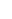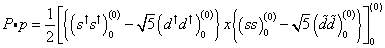(2)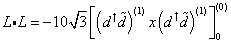(3)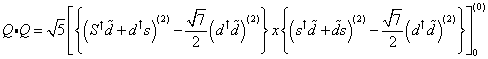(4)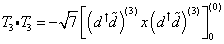(5)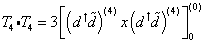(6)

In the previous formulas, nd is the number of bosons; P.P, L.L, Q.Q, T3.T3 and T4.T4 represent pairing, angular momentum, quadrupole, octupole and hexadecupole interactions between the bosons; EPS is the boson energy; and PAIR, ELL, QQ, OCT, HEX is the strengths of the pairing, angular momentum, quadrupole, octupole and hexadecupole interactions.

3. Transition Rates

The electric quadrupole transition operator employed in this study is given by: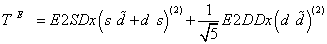(7)

where:

T(E2): absolute transition probability of the electric quadrupole (E2) transition, and E2SD and E2DD: adjustable parameters

The reduced electric quadrupole transition rates between Ii→If states are given by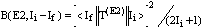(8)

where

Ii: the initial state of the electric quadrupole transition, and

If: the final state of the electric quadrupole transition.

4. Results and Discussion

4.1. The Potential Energy Surfaces

The potential energy surfaces, V(β, γ), for 154 Gd nucleus as a function of the deformation parameters β and γ has been calculated using equation:

E Nυ N (β, γ) = < N Nυ;βγ | Hnp | N Nυ; βγ >

= εd (Nυ+ N2(1+ β2)-1 +

β2(1+β2)-2 {k N Nυ[4-(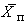+)βcos3 γ+β2] +Nυ(Nυ-1) (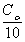+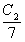) β2}            (9)

where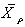= (2/7)0.5 Xρ ρ=or υ                     (10)

The calculated potential energy surfaces, V (β, γ), are Presented in Figure 1. The deviation from spherical, an harmonic vibrator U (5), to rotational characters, SU (3), at the critical symmetry point has supported quite well as well as the energy and transition probability ratios the X(5) characters to 154Gd nucleus, Table. 1.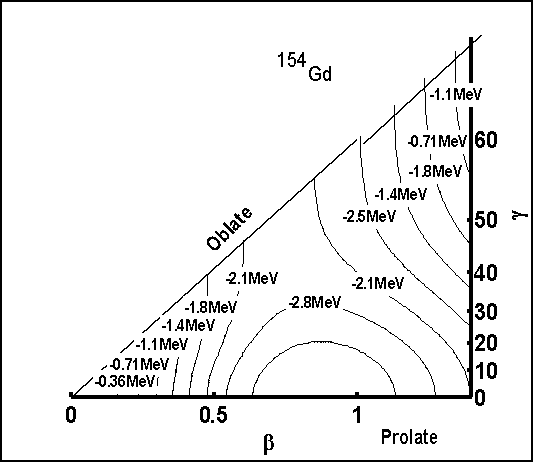Figure 1. Contour plot of the potential energy surfaces.

4.2. Energy Spectra

The energy of the positive and negative parity states of 154 Gd isotope are calculated using computer code PHINT  with the parameters EPS = 0.424, P AIR = 0.000, ELL = 0.0084, QQ = −0.0244, OCT = 0.000, HEX = 0.000 all in MeV while, E2SD =0.1450(eb), E2DD = −0.4289(eb). A comparison between the experimental spectra  and our calculations, using values of the model parameters given in Table 1 for the ground state, β1, β2, γ1, γ2 and (−ve) parity bands are illustrated in Figure 2. The agreement between the calculated levels energy and their correspondence experimental values are fairly good in low-lying states and slightly higher for the higher excited states. We believe this is due to the change of the projection of the angular momentum which is due mainly to band crossing.Figure 2. Comparison between exp.  and theo. IBA calculations.

4.3. Transition Rates

Unfortunately there is no enough measurements of electromagnetic transition rates B(E1) or B(E2) for 154 Gd nucleus. The only measured value is B(E2, 0+1 → 2+1) = 3.89(7)  which presented in Table 2. The parameters E2SD and E2DD are used in the computer code NPBEM  for calculating the electromagnetic transition rates and then normalized to the experimental value, B(E2, 0+1 → 2+1).

No new parameters are introduced for calculating electromagnetic transition rates B(E1) and B(E2) of intra band and inter band. It is clear that the electromagnetic transition of the γ transition within any band has a large value while it is small between intra bands which is due to either a mixture of M 1 or it is forbidden.

4.4. Back Bending

The moment of inertia J and energy parameters ω are calculated using Eq. (11, 12):

2J/ħ2 = (4I-2) /ΔE(I→ I-2)                   (11)

(ħω)2 = (I2 – I + 1)[ΔE(I→ I-2)/(2I-1)]        (12)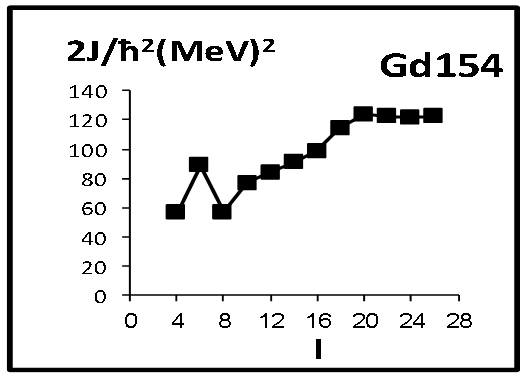Figure 3. Back bending.

Table 1. Energy and transition probability ratios.

 Nucleus E41+/E21+ E61+/E21+ E81+/E21+ E02+/E21+ E61+/E02+ E03+/E21+ BE2(41+-- 21+) / BE2(21+-- 01+) 154Gd 3.00 5.83 9.30 5.52 1.05 9.60 1.52 X(5) 3.02 5.83 9.29 5.65 1.53 6.03 1.58

Figure 3. shows forward bending for154 Gd at I + = 6 and that bending has explained as due to partial rotational alignment of a pair of neutrons near the Fermi surface.

Table 2. Calculated B(E2) and B(E1).

 Ii+ If+ B(E2) Exp*. B(E2) IBA Ii+ If+ B(E1) Exp. B(E1) IBA 01 21 3.89(7) 3.8965 11 01 —— 0.1087 22 01 —— 0.0005 11 02 —— 0.0786 22 02 —— 0.4922 31 21 —— 0.2465 23 01 —— 0.0168 31 22 —— 0.0826 23 02 —— 0.0123 31 23 —— 0.0083 23 03 —— 0.0498 32 21 —— 0.0352 24 03 —— 0.3081 32 22 —— 0.0214 24 04 —— 0.0358 32 23 —— 0.0564 22 21 —— 0.0628 51 41 —— 0.3710 23 21 —— 0.0165 51 42 —— 0.0733 23 22 —— 0.4976 51 43 —— 0.0043 41 21 —— 1.1878 71 61 —— 0.4943 41 22 —— 0.0694 71 62 —— 0.0631 61 41 —— 1.3454 91 81 —— 0.6193 61 42 —— 0.0587 91 82 —— 0.0545 81 61 —— 1.4041 111 101 —— 0.7469 81 62 —— 0.0467 81 63 —— 0.0234 101 81 —— 1.3954

Ref.Figure 4. Beat pattern.

4.5. The Staggering

The presence of odd-even parity states has encouraged us to study staggering eect for 154Gd. Staggering pattern between the energies of the ground state and the (−ve) parity octupole bands have been calculated, ∆I = 1, using staggering function Eq. (13, 14) with the help of the available experimental data .

Stag (I) = 6∆E(I) − 4∆E(I − 1) − 4∆E(I + 1) + ∆E(I + 2) +∆E(I − 2) (13)

with

∆E(I) = E(I + 1) − E(I)                     (14)

The calculated staggering pattern has illustrated in Figure 4. It shows an interaction between the (−ve) and (+ve) parity states.

4.6. Electric Monopole Transitions, Xif ′ f (E0/E2)

The electric monopole transitions, E0, are normally occurring between two states of the same spin and parity by transferring energy and zero unit of angular momentum. The strength of the electric monopole transition, Xif ′ f (E0/E2),  can be calculated using Eq. (15, 16) and results are presented in Table 3.

Table 3. Calculated Xif f (E0/E2)

 Ii+ If+ I'f+ Xif ′ f (E0/E2)Exp* Xif ′ f (E0/E2)IBA 02 01 21 ------ 5.11 03 01 22 ------ 0.67 03 02 22 ------ 3.11 04 01 23 ------ 1.13 04 02 23 ------ 0.67 04 03 23 ------ 4.54 23 22 21 ------ 1.04 24 22 21 ------ 6.28 24 23 21 ------ 1.77

The small values of the Xif ′ f (E0/E2) indicate that the transition has small contribution of E0 transition, while the large valeue means that the decay of the state is mainly E0 which is by turn responsible for the shape of the nucleus.

Xif ′ f (E0/E2) = B(E0, Ii – If) / B(E2, Ii – I'f)     (15)

Where

Ii = If = 0, I'f =2 or Ii = If ≠ 0, If = I'f

Xif ′ f (E0/E2 = 2.54x109 A3/4 [E5γ MeV/ ΩKL]x α (E2) x[Te(E0, Ii – If) / Te(E2, Ii – I'f)]     (16)

A: mass number; depopulating it;

If: spin of the ﬁnal state of E0 transition;

I′ f: spin of the ﬁnal state of E2 transition;

Eγ: gamma ray energy;

KL: electronic factor for K, L shells ;

α(E2): conversion coecient of the E2 transition;

Te (E0, Ii − If): absolute transition probability of the E0 transition

between Ii and If states, and

Te (E2, Ii − I′ f): absolute transition probability of the E2 transition between Ii and I′ f states.

5. Conclusions

IBA-1 model has been applied successfully to 154Gd isotope and:

1. The levels energy are successfully reproduced;

2. The levels energy, transition probability ratios and potential energy surfaces are calculated and show X(5) characters to 154Gd;

3. Electromagnetic transition rates B(E1) and B(E2) are calculated;

4. Forward bending has observed at angular momentum I+ = 6;

5. Strength of the electric monopole transitions Xif ′ f (E0/E2) are calculated; and

6. Staggering eect has been calculated and beat pattern has obtained which show an interaction between the (−ve) and (+ve) parity states.

References

1. J.Beller, N. Pietralla, J. Barea, M. Elvers, J. Endres, C.Fransen, J. Kotila, O. Moller, A. Richter, T. R. Rodriguez, C.Romig, D. avran, M. Scheck, L. Schnorrenberger, K.Sonnabend, V. Werner, A. Zilges, and M. weidinger., "Constraint on 0νββ Matrix Elements from a Novel DecayChannel of the Scissors Mode: The Case of 154Gd",Phys.Rev. Lett.,V.111, 172501, (2013).
2. Wen-Te Liao, Adriana Paly, and Christoph H. Keitel, Three-beam setup for coherently controlling nuclear-state popula-tion",Phys. Rev. C 87, 054609,(2013).
3. N. D. Scielzo, J. E. Escher, J. M. Allmond, M. S. Basunia,C. W. Beausang, L. A. Bernstein, D. L. Bleuel, J. T. Burke,R. M. Clark, F. S. Dietrich, P. Fallon, J. Gibelin, B. L. Gold-blum, S. R. Lesher, M. A. McMahan, E. B. Norman, L. Phair,E. Rodriguez-Vieitez, S. A. Sheets, I. J. Thompson, and M.Wiedeking,"Statistical γ rays in the analysis of surrogate nu-clear reactions",Phys. Rev. C 85, 054619,(2012).
4. J. F. Sharpey-Schafer, S. M. Mullins, R. A. Bark, J. KauF. Komati, E. A. Lawrie, J. J. Lawrie, T. E. Madiba,P. Maine and 4 more,"Congruent band structures in 154Gd:Conﬁguration-dependent pairing, a double vacuum andlack ofβ -vibrations",The European Physical Journal A,47, 5,(2011).
5. J. F. Sharpey-Schafer, T. E. Madiba, S. P. Bvumbi, E. A.Lawrie, J. J. Lawrie, A. Minkova, S. M. Mullins, P. Papka,D. G. Rouxand 1 more,"Blocking of coupling to the 0+ ex-2citation in 154Gd by the 11/2− neutron in 155 Gd",TheEuropean Physical Journal A, 47, 6,(2011).
6. N. D. Scielzo, J. E. Escher, J. M. Allmond, M. S. Basunia,C. W. Beausang, L. A. Bernstein, D. L. Bleuel, J. T. Burke,R. M. Clark, F. S. Dietrich, P. Fallon, J. Gibelin, B. L. Gold-blum, S. R. Lesher, M. A. McMahan, E. B. Norman, L. Phair,E. Rodriquez-Vieitez, S. A. Sheets, I. J. Thompson, and M.Wiedeking,"Measurement of γ-emission branching ratios forGd154,156,158 compound nuclei: Tests of surrogate nuclearreaction approximations for (n,γ) cross sections",Phys. Rev. C81, 034608,(2010).
7. N. D. Scielzo, L. A. Bernstein, D. L. Bleuel, J. T. Burke1, S.R. Lesher, E. B. Norman, S. A. Sheets, M. S. Basunia, R. M.Clark, P. Fallon, J. Gibelin, B. Lyles, M. A. McMahan, L. G.Moretto, L. W. Phair, E. Rodriguez-Vieitez, M. Wiedeking, J.M. Allmond and C. W. Beausang,"Determininig the (n, γ)(n, γ)cross section of 153Gd using surrogate reactions",AIPConf. Proc., 1005,109, (2008).
8. J.F. Sharpey-Schafer, S. M. Mullins, R. A. Bark, E. Gue-orguieva, J. Kauc, F. Komati, J. J. Lawrie, P. Maine, A.Minkova, S. H. T. Murray, N. J. Ncapayi and P. Vymers,"Shape Transitional Nuclei: What can we learn from the YrareStates? or Hello the Double Vacuum; Goodbye β-vibrations!",AIP Conf. Proc., 1012, 19 (2008).
9. A. Dewald, O. Moller, D. Tonev, A. Fitzler, B. Saha, K.Jessen, S. Heinze, A. Linnemann, J. Jolie and 19 more,"ShapeChanges and test of the critical-point symmetry X(5) in N = 90nuclei",The European Physical Journal A,20,173,(2003).
10. W. D. Kulp, J. L. Wood, K. S. Krane, J. Loats, P. Schmelzen-bach, C. J. Stapels, R.-M. Larimer, and E. B. Norman,"N=90region: The decay of Eu154 to Gd154",Phys. Rev. C 69,064309, (2004).
11. Buganu1 P and Budaca R,"Sextic potential for γ -rigid prolatenuclei",Journal of Physics G, V. 42, 105201,(2015).
12. H. Sabri,"Spectral statistics of rare-earth nuclei: Investigationof shell model conﬁguration eect",Nuclear PhysicsA,941,364, (2015).
13. E. Ganioglu, R. Wyss, and P. Magierski,"Properties of N=90isotones within the mean ﬁeld perspective",Phys. Rev. C 89,014311,(2014).
14. J.B. Gupta,"Test of the Grodzins product rule in N = 88 iso-tones and the role of the Z = 64 subshell",Phys. Rev. C 89,034321,(2014).
15. Nikolay Minkov and Phil Walker,"Inﬂuence of the octupolemode on nuclear high-K isomeric properties",The RoyalSwedish Academy of Sciences, Physica Scripta, Volume 89,Number 5,(2014).
16. Harun Resit Yazar,"Low-lying (K π =0+) states ofGadolinium isotopes",Pramana J. Phys.,81, 579, (2013).
17. J.Kotila, K. Nomura, L. Guo, N. Shimizu, and T. Ot-suka,"Shape phase transitions in the interacting boson model:Phenomenological versus microscopic descriptions",Phys.Rev. C 85, 054309, (2012).
18. N. Minkov, S. Drenska, M. Strecker, W. Scheid, and H.Lenske,"Non-yrast nuclear spectra in a model of coherentquadrupole-octupole motion",Phys. Rev. C 85, 034306,(2012).
19. N. Minkov and Phil Walker,"Magnetic moments of K isomersas indicators of octupole collectivity",The European PhysicalJournal A,48, 80, (2012).
20. M. S.Nadirbekov, G. A. yuldasheva,N. Minkov and W.Scheid,"Collective excited states in even-even nuclei withquadrupole and octupole deformations",Int. J. Mod. Phys. E21, 1250044, (2012).
21. Dai Lian-Rong, Teng Wei-Xin, Pan Feng and WangSheng-Hua,"An Alternative Interacting Boson ModelDescription of The N = 90 Nuclei",Chinese Physics Letters,28, 052101,(2011).
22. L.M.Robledo, R. R. Rodriguez-Guzmon, and P. Sar-riguren,"Evolution of nuclear shapes in medium mass iso-topes from a microscopic perspective",Phys. Rev. C 78,034314,(2008).
23. G.Puddu, O. Scholten, T. Otsuka,"Collective QuadrupoleStates ofXe, Ba and Ce in the Interacting Boson Model",Nucl.Phys. A, 348, 109, (1980).
24. A.Arima and F. Iachello"Interacting boson model ofCollective states: The vibrational limit.",Ann. Phys., 99, 253,(1976).
25. A.Arima and F. Iachello,"Interacting boson model of collec-tive states: The rotational limit", Ann. Phys.,111, 201, (1978).
26. Arima A. and Iachello F.,"Interacting boson model of collec-tive states: The O(6) limit.", Ann. Phys., 123, 468, (1979).
27. J.N. Ginocchio and M. W. Kirson,"An intrinisic state forthe in]teracting boson model and its relationship to the Bohr-Mottelson approximation", Nucl. Phys. A, 350, 31, (1980).
28. Scholten O.,"The programm package PHINT (1980)version", internal report KVI-63, Gronigen: KeryfysischVersneller In stitut 1979.
29. C.W. Reich,"Adopted levels, gammas for 154Gd",NuclearData Sheets 110, 2257 (2009).
30. B.Pritchenko, M. Birch, B. Singh and M. Horoi, "Tables ofE2 transition probabilities from the ﬁrst 2+ states in even-Even nuclei", Atom. Dat. Nucl. Tab., 107, 1-139, (2016).
31. J.O.Rasmussen,"Theory of E0 transitions of spheroidal nu-clei." Nucl.Phys., 19, 85, (1960).
32. A.D. Bell, C. E. Aveldo, M. G. Davidson and J. P. Davidson"Table of E0 conversion probability electronic factors.", Can.J. Phys., 48, 2542, (1970).

 Contents 1. 2. 3. 4. 4.1. 4.2. 4.3. 4.4. 4.5. 4.6. 5.
Article ToolsAbstractPDF(274K)